Courses

# Explanation and Solved Examples GMAT Notes | EduRev

## Quantitative Aptitude for GMAT

Created by: Wizius Careers

## GMAT : Explanation and Solved Examples GMAT Notes | EduRev

The document Explanation and Solved Examples GMAT Notes | EduRev is a part of the GMAT Course Quantitative Aptitude for GMAT.
All you need of GMAT at this link: GMAT

Definition: A circle is a simple closed figure in which all the points that lie on it are equidistant from a point called center
Here’s a circle with center O.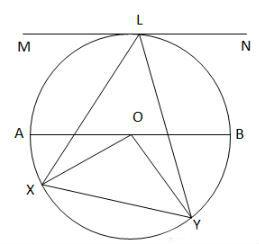Here are some basics regarding circle and its properties:

Radius: The line segment that connects the center of the circle to any point on the circle is called radius. OB, OA, OX, OY are the different radii of the circle in the figure above. Infinite number of radii can be drawn for any circle.

Diameter: The line segment that passes through the center and connects two points on the circle is called the diameter. AB is the diameter of the circle. Like radius, infinite number of diameters can be drawn in a circle.
Diameter = 2 * radius Or

Chord: The line segment that connects any two points on a circle is a chord. Infinite number of chords can be drawn in a circle. XY is a chord of the circle.
Apparently, the diameter is the longest chord of a circle.

Circumference: The measure of the distance around the edge of a circle is the circumference of the circle.
The circumference of a circle is given by the formula,
C = 2pr
Where C is the circumference and r is the radius.
Since 2*radius = diameter, Circumference is also given by
C = pd
The value of p is approximately 22/7 or 3.14159.

Area:
The area of a circle is given by the formula,
A = pr2
Where r is the radius of the circle.
Since r=d/2, Area is also given by
A = pd2/4

Arcs and sectors:
An arc is a part of the circumference of the circle. XY is an arc in the figure above.
A sector is the area covered by two radii and the arc connecting them. XLY and XOY are two of the sectors in the figure above.
Length of an arc is given by the formula, (x/360) * 2pr
Area of a sector is given by the formula, (x/360) * pr2
Where x is the angle subtended by the arc and r is the radius.
Central Angle: The angle whose one vertex lies on the center of the circle is a central angle. ∠XOY is a central angle in the figure above.
Inscribed angle: The angle whose one vertex lies on one part of the circle and the other two end points lie on other place on the circle, is called an inscribed angle. ∠XLY, ∠OXY, ∠OYX are some of the inscribed angles in the figure above.
Inscribed angles subtended by the same arc are equal.
A central angle is twice the corresponding inscribed angle.
In the figure above,
∠XOY = 2 ∠XLY
Tangent: A tangent of a circle is a line that just touches the circle at one point without intersecting it. MN is the tangent in the figure above.
Circumscribed circle: If a polygon is present inside a circle in such a way that all its vertices lie on the circle, or just touch the circle, then the circle is called a circumscribed circle.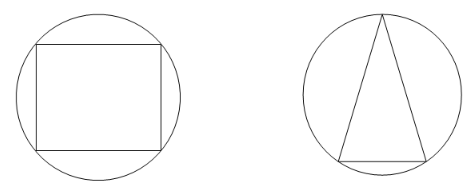Note: When a triangle is inscribed inside a circle and if one of the sides of the triangle is diameter of the circle, then the diameter acts as hypotenuse and the triangle is right. This is called the Thales’ theorem.

Inscribed circle: If a circle is present inside a polygon in such a way that the sides of polygon are just touching the circumference of the circle then the circle is called an inscribed circle.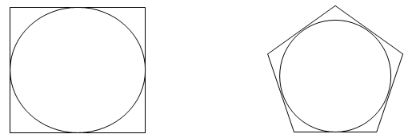Semicircle: A semi-circle is half the circle.
Area of a semicircle = pr2 / 2
Circumference of a semicircle = Half the perimeter of a circle + length of the diameter
→ (2pr/2) + 2r
→ pr+2r
→ r(2+p)

Solved examples involving Circles
Problem 1: Area of the circle above is 81p. ∠DAB = 55°. Find the central angle subtended by arc DB and the area and circumference of the sector DOB.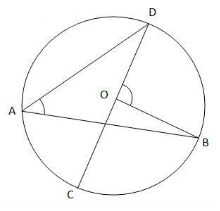Solution:
Area of the circle = pr2 = 81p
→ r2 = 81 and r = 9.
∠DAB is the inscribed angle subtended by the arc DB. Inscribed angle is half the central angle.
∠DAB = 55° → ∠DOB = 110°.
Area of the sector DOB = (110/360)*81p = 24.75 p
Circumference of the sector is the length of the two radii + length of the arc DB
Length of the arc DB = (110/360)*2*p*9 = 5.5 p
Circumference of the sector DOB = 9 + 9 + 5.5p = 18 + 5.5p

Problem 2: If QS is the diameter and QR = 6 and radius = 5, find the area of the shaded region.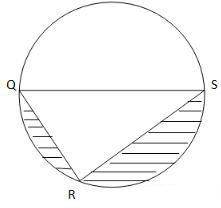Solution:
Area of the shaded region = Area of the circle – (Area of the triangle QRS + Area of the semicircle)
Area of the circle = 25p
Area of the semicircle = 12.5p
Finding the area of the triangle:
According to the Thales’ theorem, if diameter is the side of a triangle, then it becomes the hypotenuse and the triangle is right.
Therefore, ∠QRS = 90°.
Radius = 5 → Diameter, QS = 10
QR = 6 (given)
According to Pythagoras theorem, QS2 = QR2 + RS2
100 = 36 + RS2 → RS = 8. We can also see that this triplet is a multiple of the famous Pythagorean triplet 3, 4, 5.
Area of a triangle = base * height / 2 = 48 / 2 = 24
Area of the shaded region = 25p – (24 + 12.5p) = (12.5p – 24) sq. units.

Offer running on EduRev: Apply code STAYHOME200 to get INR 200 off on our premium plan EduRev Infinity!

## Quantitative Aptitude for GMAT

85 videos|100 docs|176 tests

,

,

,

,

,

,

,

,

,

,

,

,

,

,

,

,

,

,

,

,

,

;